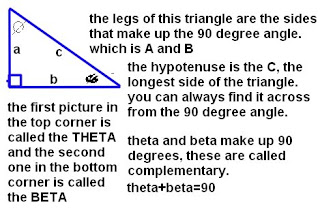### Scribe Post for February 20

Wednesday, February 25, 2009
Since no one's doing the scribe post for February 20, I'll just do it. It sucks to have an empty Math Blog when the other grade eight classes are filled with work. Geez guys. By looking at 873's scribe for February 20, I remembered what we learned that day. Thank you reNz873!

On February 20, we talked about the Pythagorean Theorem

TriangleThis is the triangle. The sides that make up the 90 degree angle are called legs and are labelled as A AND B. The hypotenuse is the C, and is the longest side of the triangle. You can always find it across from the 90 degree angle. Theta and Beta and the pictures show at the top corner and the bottom corner. The top one is the Theta and the bottom one is the Beta. Theta and Beta equal 90 degrees, which are called complementary angles.

This is a square, all the sides are equal. There are four 90 degree angles in this shape, which equals up to 360 degrees. (4x90=360) You can easily find the area or perimeter of the square if you know the measurement for one side. Example shown in the picture. One side is 5, so we know that all sides equal 5. 5x5, lxw, the area is 25. 5x5=25. Length-5, Width-5.Pythagorean Theorem
This is the the pythagorean theorem. The first square is 3x3, the second square is 4x4 and the last square is a combination of both squares, 5x5. You get to the last one, by putting the yellow square in the inside of the 5x5 square. It all fits in there, there is a little space left for the 3x3 square. So, you place it on there.

9+16=25
3x3+4x4=5x5

I hope this is what we learned! Sorry if it's not, the 873 one was the one scribe I could find. So, I'm going to pick someone who'll actually do it. Arielle. :) Love you too Arielle.